# 圖解OneFlow的學習率調整策略1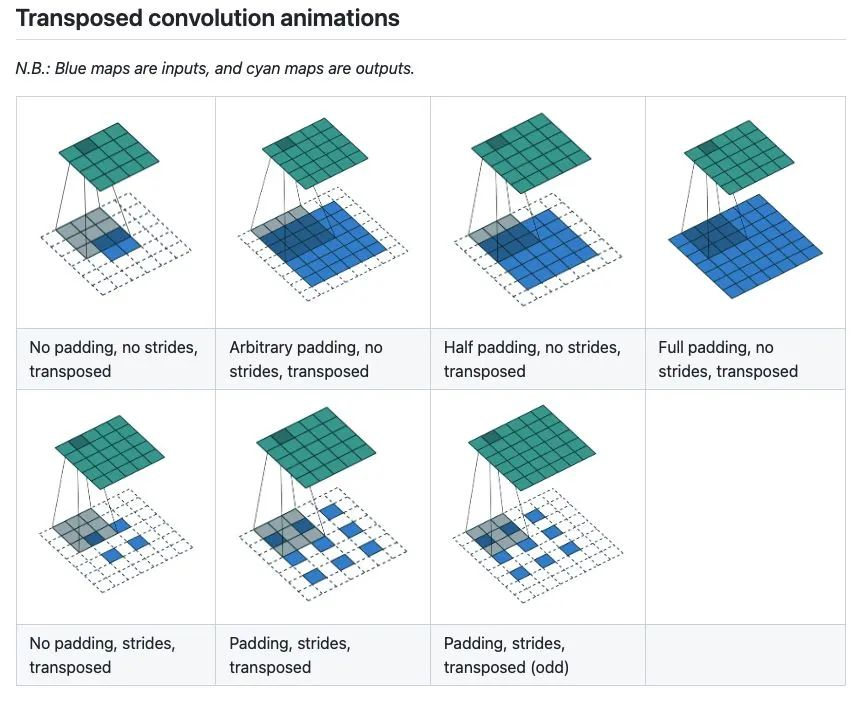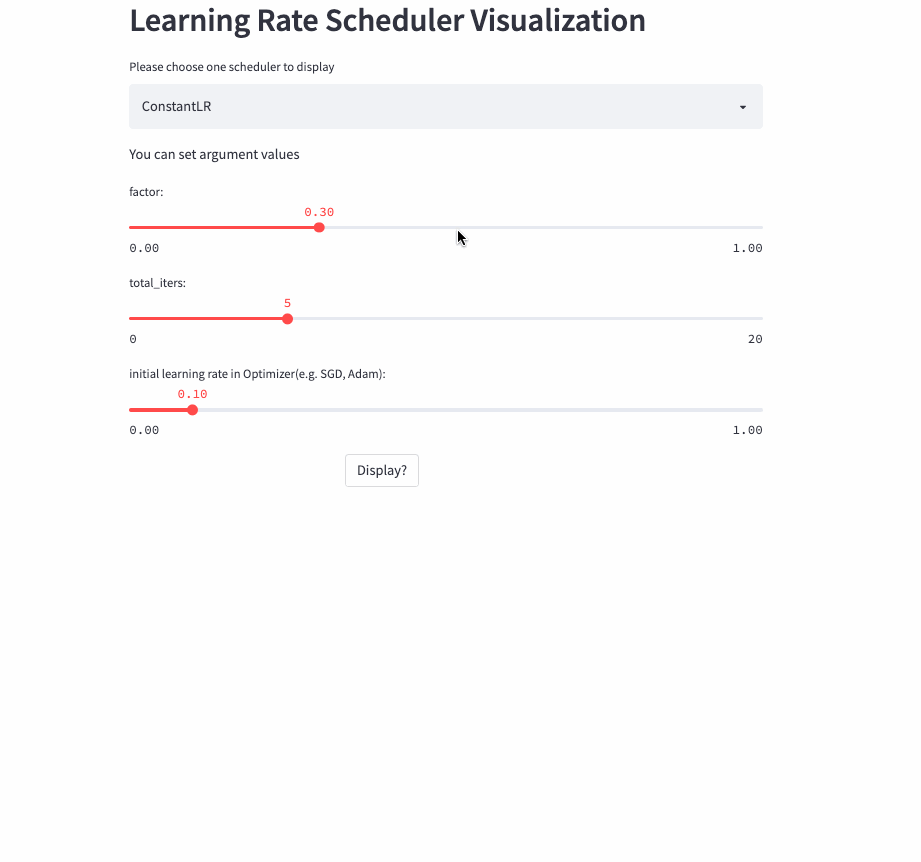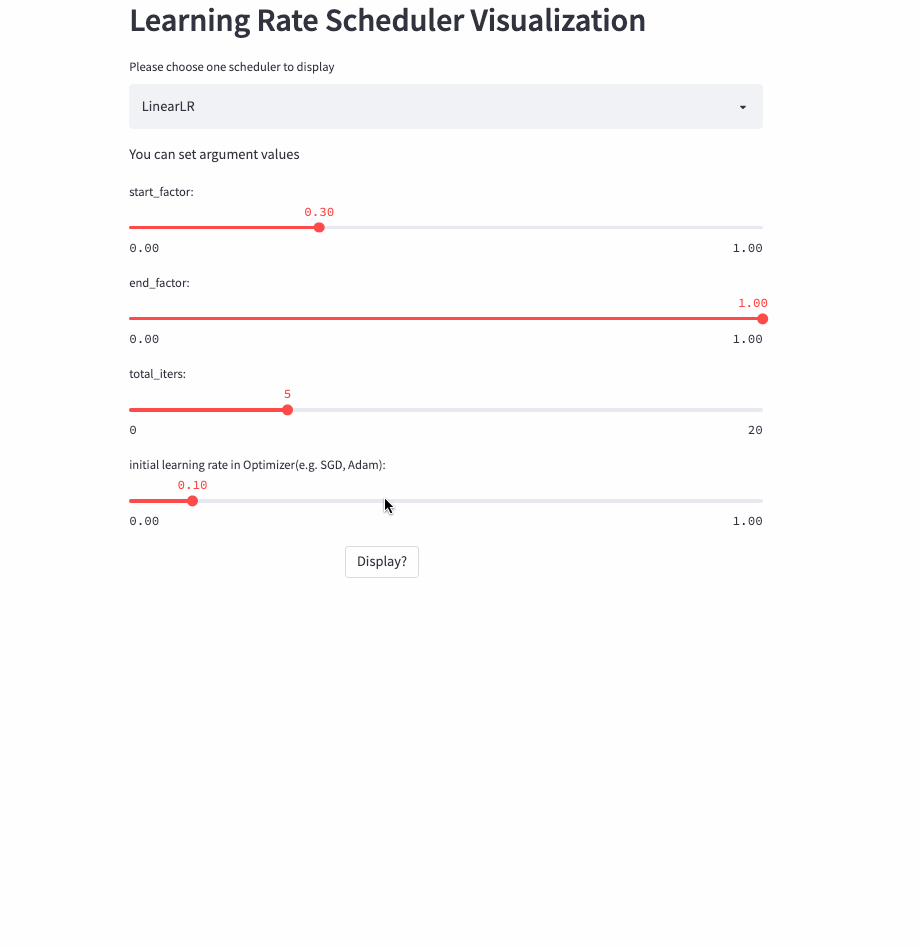• https://huggingface.co/spaces/basicv8vc/learning-rate-scheduler-online

• https://share.streamlit.io/basicv8vc/scheduler-online

## 學習率調整策略

### 基類LRScheduler

LRScheduler(optimizer: Optimizer, last_step: int = -1, verbose: bool = False)是所有學習率排程器的基類，初始化引數中last_step和verbose一般不需要設定，前者主要和checkpoint相關，後者則是在每次step() 呼叫時列印學習率，可以用於 debug。LRScheduler中最重要的方法是step()，這個方法的作用就是修改使用者設定的初始學習率，然後應用到下一次的Optimizer.step()。

### ConstantLR

``oneflow.optim.lr_scheduler.ConstantLR(``    optimizer: Optimizer,``    factor: float = 1.0 / 3,``    total_iters: int = 5,``    last_step: int = -1,``    verbose: bool = False,``)``

ConstantLR和固定學習率差不多，唯一的區別是在前total_iters，學習率為初始學習率 * factor。### LinearLR

``oneflow.optim.lr_scheduler.LinearLR(``    optimizer: Optimizer,``    start_factor: float = 1.0 / 3,``    end_factor: float = 1.0,``    total_iters: int = 5,``    last_step: int = -1,``    verbose: bool = False,``)``

LinearLR和固定學習率也差不多，唯一的區別是在前total_iters，學習率先線性增加或遞減，然後再固定為初始學習率 * end_factor。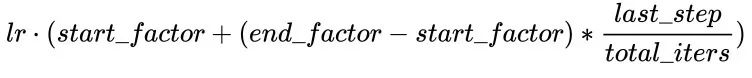LinearLR

### ExponentialLR

``oneflow.optim.lr_scheduler.ExponentialLR(``    optimizer: Optimizer,``    gamma: float,``    last_step: int = -1,``    verbose: bool = False,``)``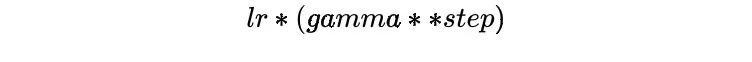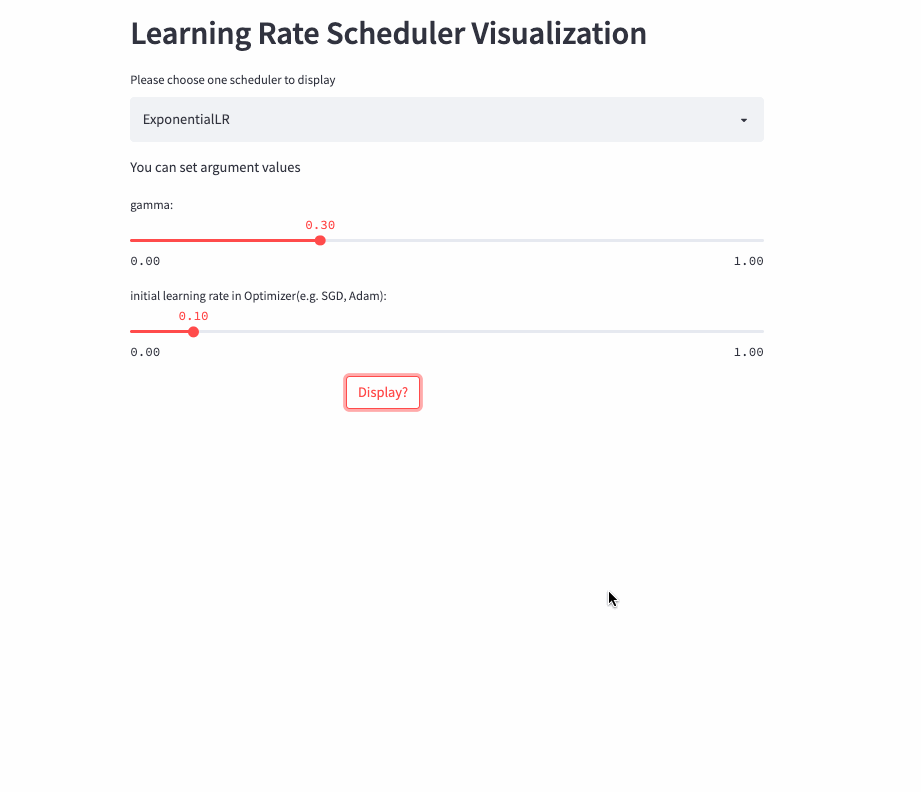ExponentialLR

### StepLR

``oneflow.optim.lr_scheduler.StepLR(``    optimizer: Optimizer,``    step_size: int,``    gamma: float = 0.1,``    last_step: int = -1,``    verbose: bool = False,``)``

StepLR和ExponentialLR差不多，區別是不是每一次呼叫step()都進行學習率調整，而是每隔step_size才調整一次。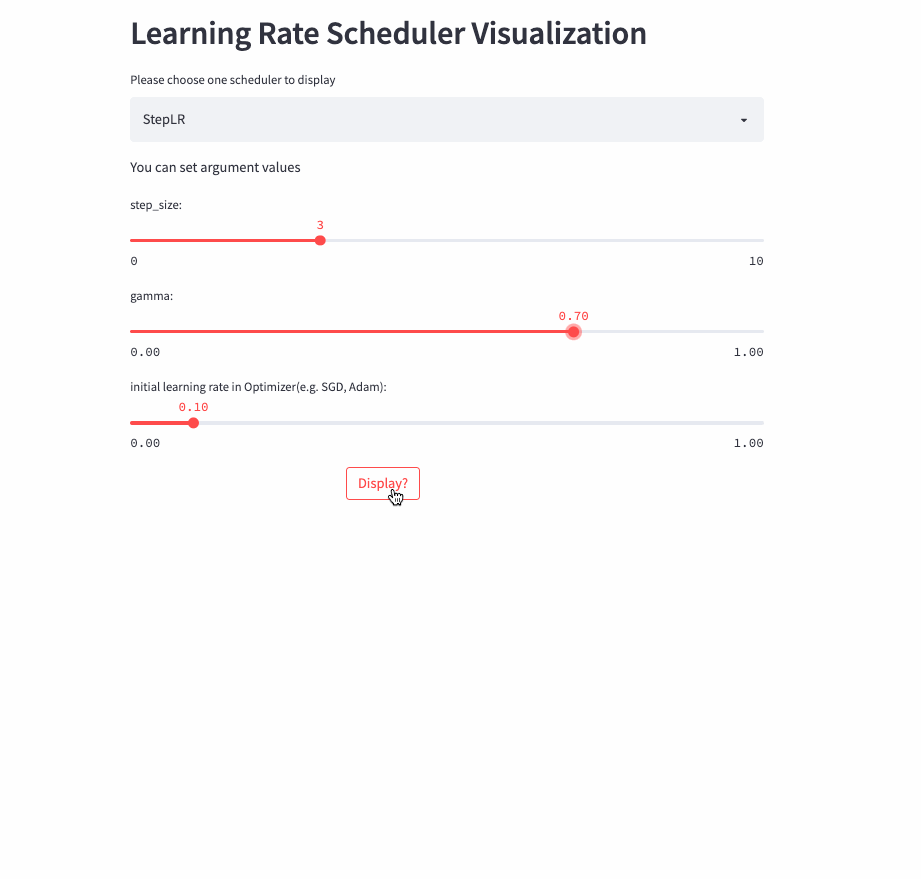StepLR

### MultiStepLR

``oneflow.optim.lr_scheduler.MultiStepLR(``    optimizer: Optimizer,``    milestones: list,``    gamma: float = 0.1,``    last_step: int = -1,``    verbose: bool = False,``)``

StepLR每隔step_size就調整一次學習率，而MultiStepLR則根據使用者指定的milestones進行調整，假設milestones是[2, 5, 9]，在[0, 2)是lr，在[2, 5)是lr * gamma，在[5, 9)是lr * (gamma **2)，在[9, )是lr * (gamma **3)。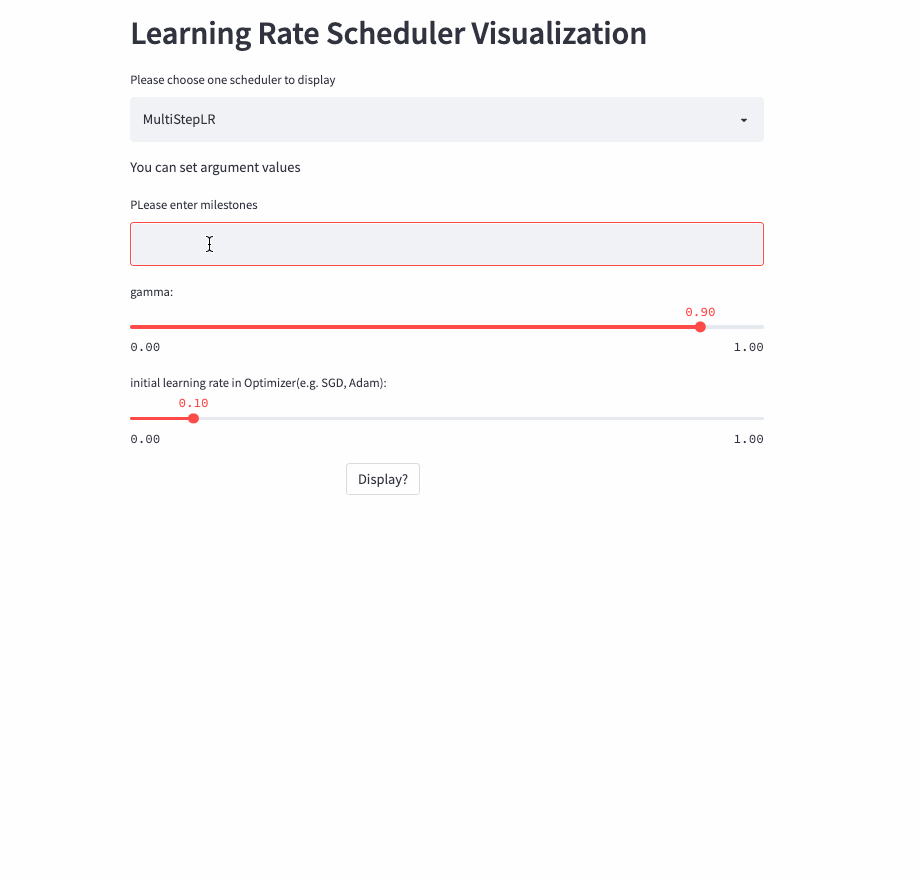MultiStepLR

### PolynomialLR

``oneflow.optim.lr_scheduler.PolynomialLR(``    optimizer,``    steps: int,``    end_learning_rate: float = 0.0001,``    power: float = 1.0,``    cycle: bool = False,``    last_step: int = -1,``    verbose: bool = False,``)``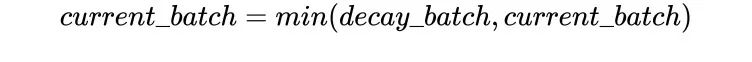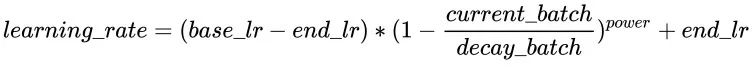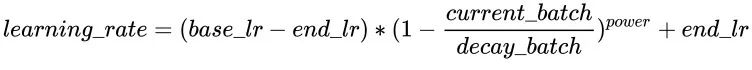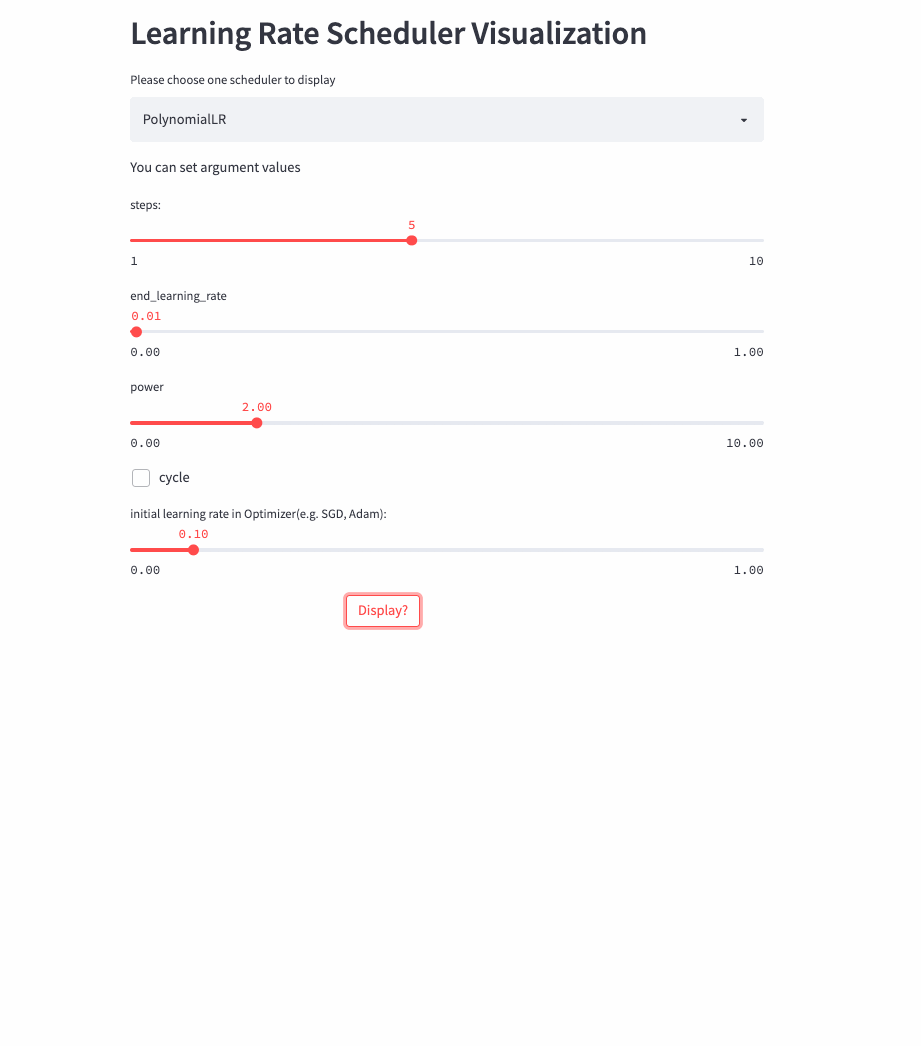PolynomialLR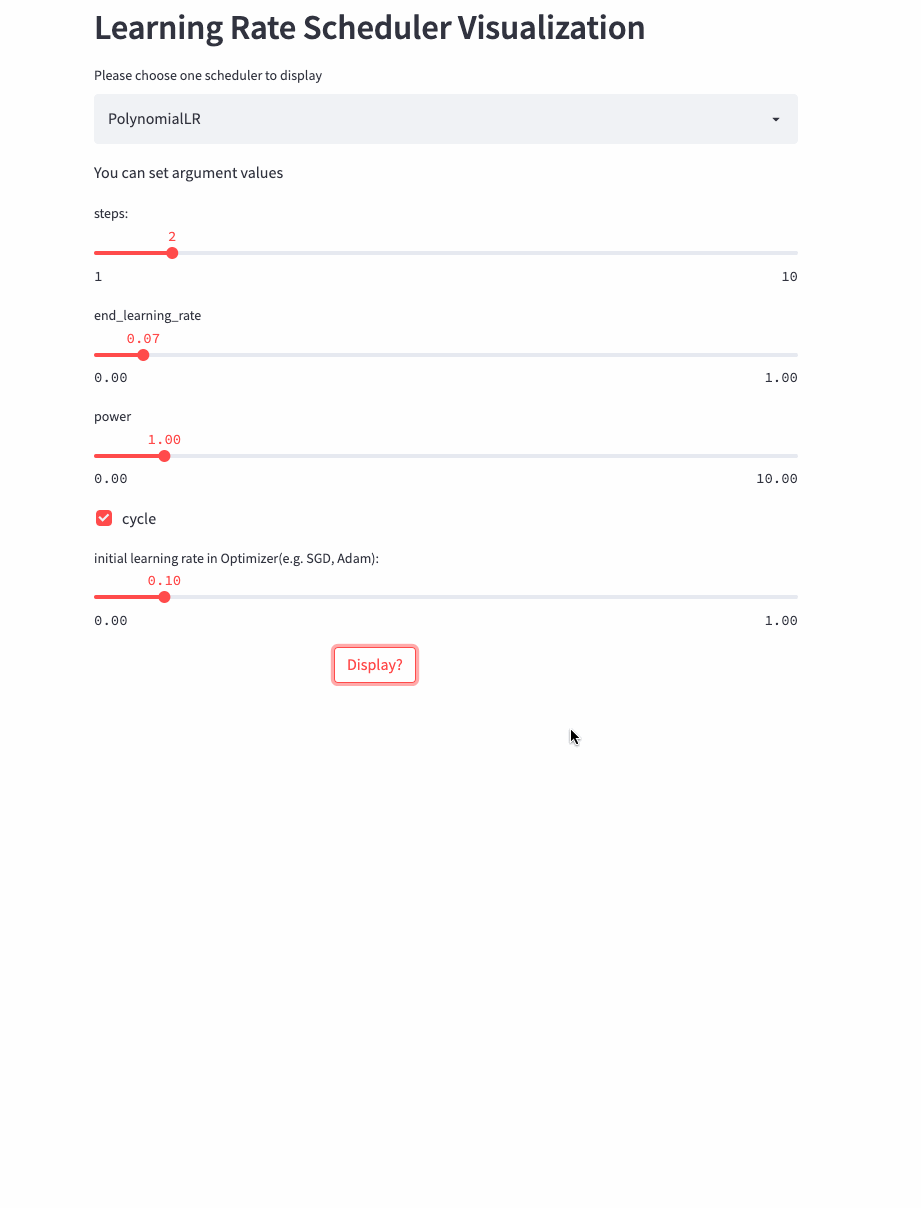### CosineDecayLR

``oneflow.optim.lr_scheduler.CosineDecayLR(``    optimizer: Optimizer,``    decay_steps: int,``    alpha: float = 0.0,``    last_step: int = -1,``    verbose: bool = False,``)``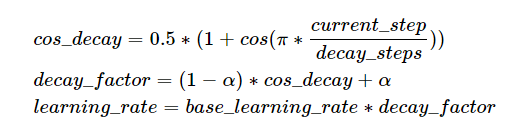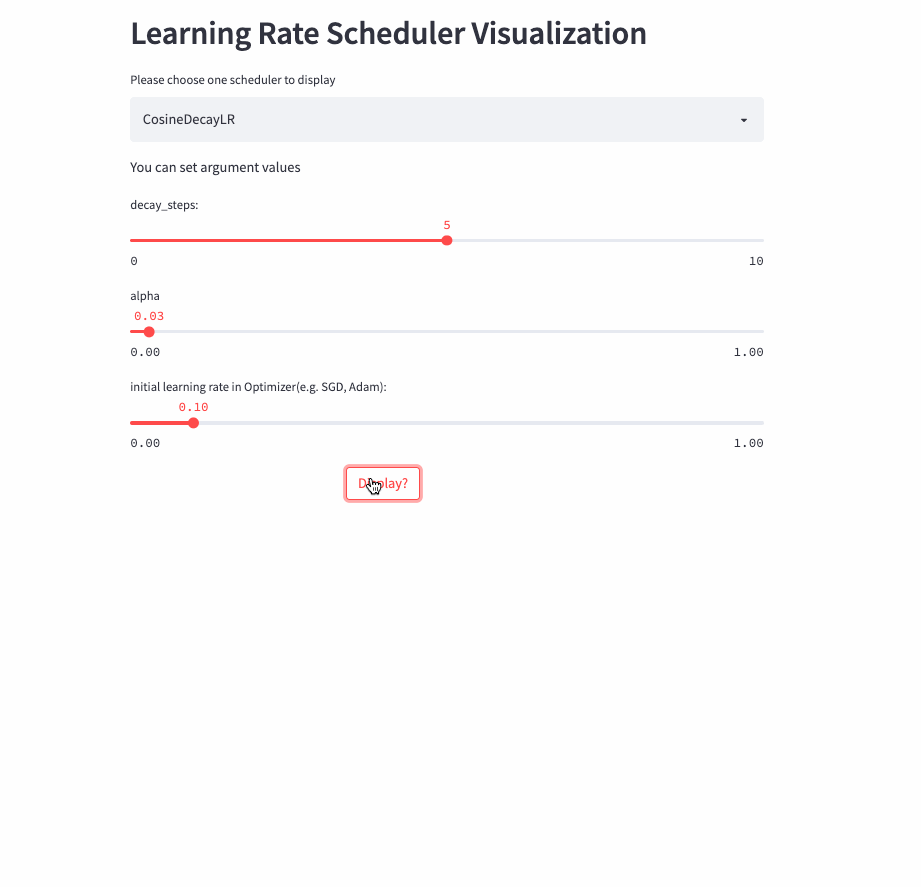### CosineAnnealingLR

``oneflow.optim.lr_scheduler.CosineAnnealingLR(``    optimizer: Optimizer,``    T_max: int,``    eta_min: float = 0.0,``    last_step: int = -1,``    verbose: bool = False,``)``

CosineAnnealingLR和CosineDecayLR很像，區別在於前者不僅包含餘弦衰減的過程，也可以包含餘弦增加，在前T_max步，學習率由lr餘弦衰減到eta_min, 如果cur_step > T_max，然後再餘弦增加到lr，不斷重複這個過程。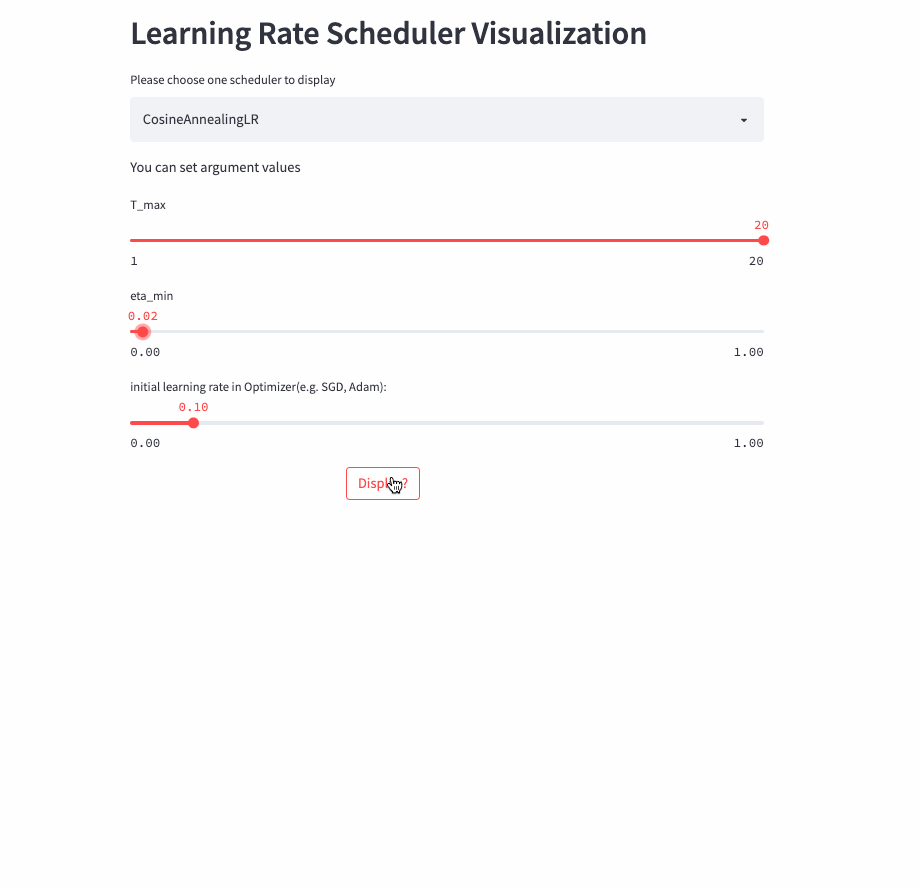CosineAnnealingLR

### CosineAnnealingWarmRestarts

``oneflow.optim.lr_scheduler.CosineAnnealingWarmRestarts(``    optimizer: Optimizer,``    T_0: int,``    T_mult: int = 1,``    eta_min: float = 0.0,``    decay_rate: float = 1.0,``    restart_limit: int = 0,``    last_step: int = -1,``    verbose: bool = False,``)``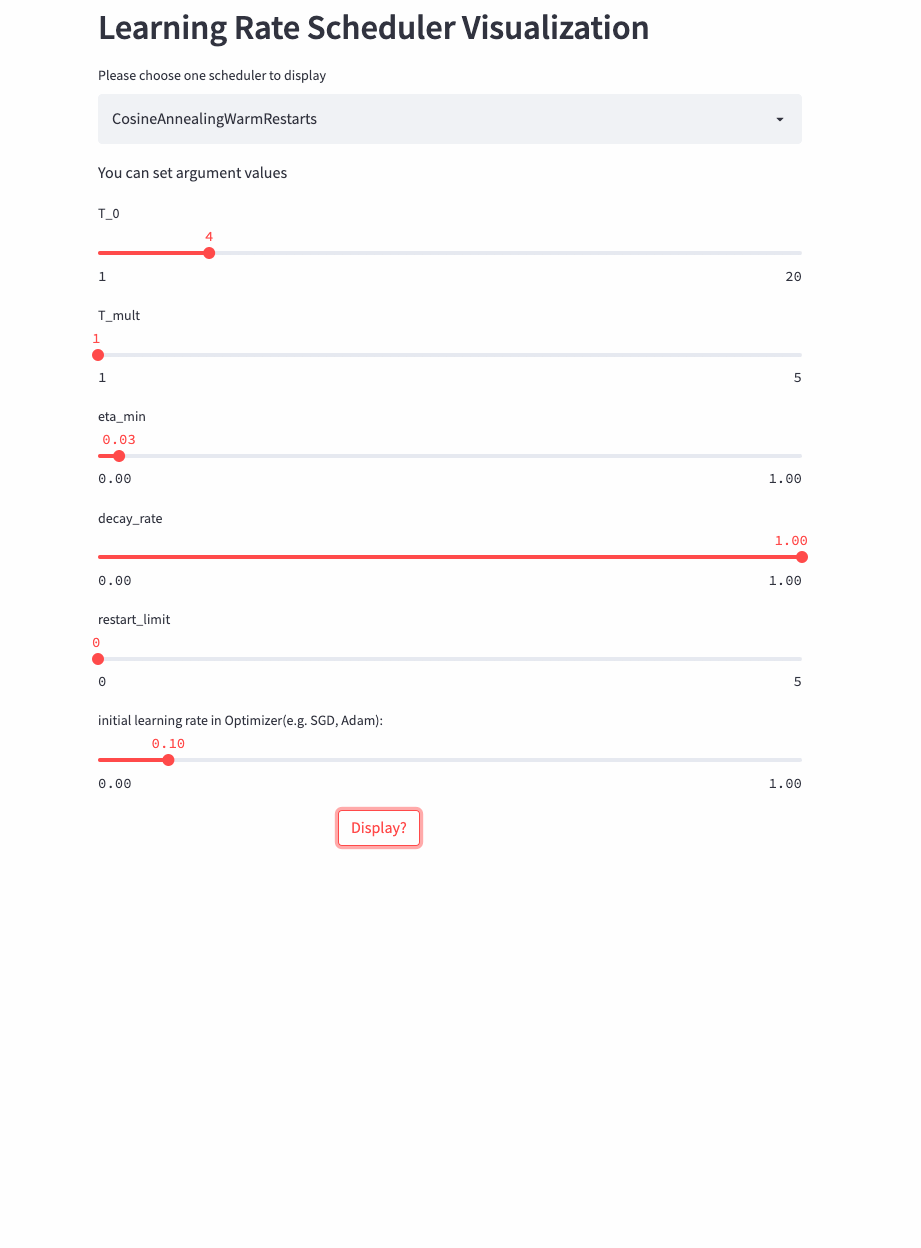T_mult=1, decay_rate=1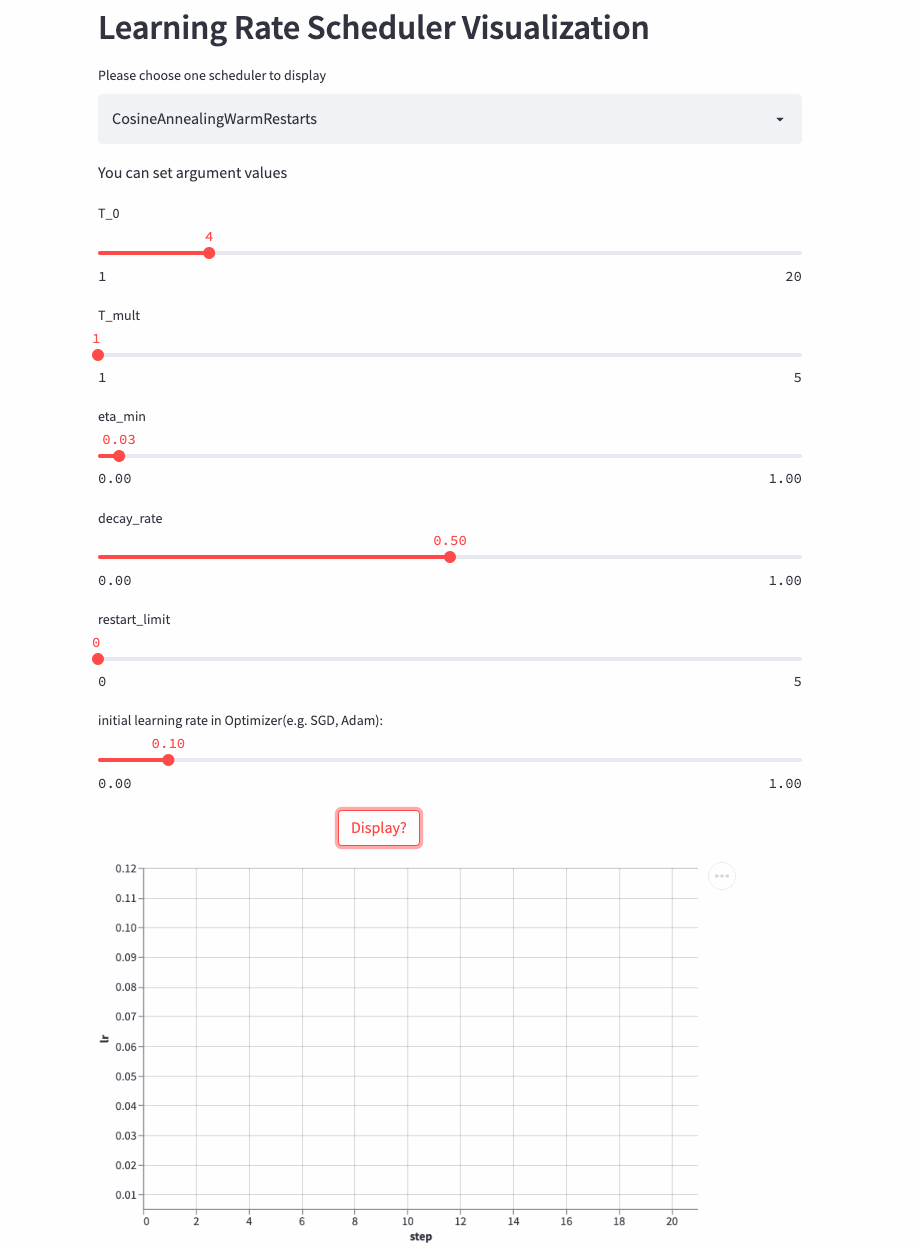T_mult=1, decay_rate=0.5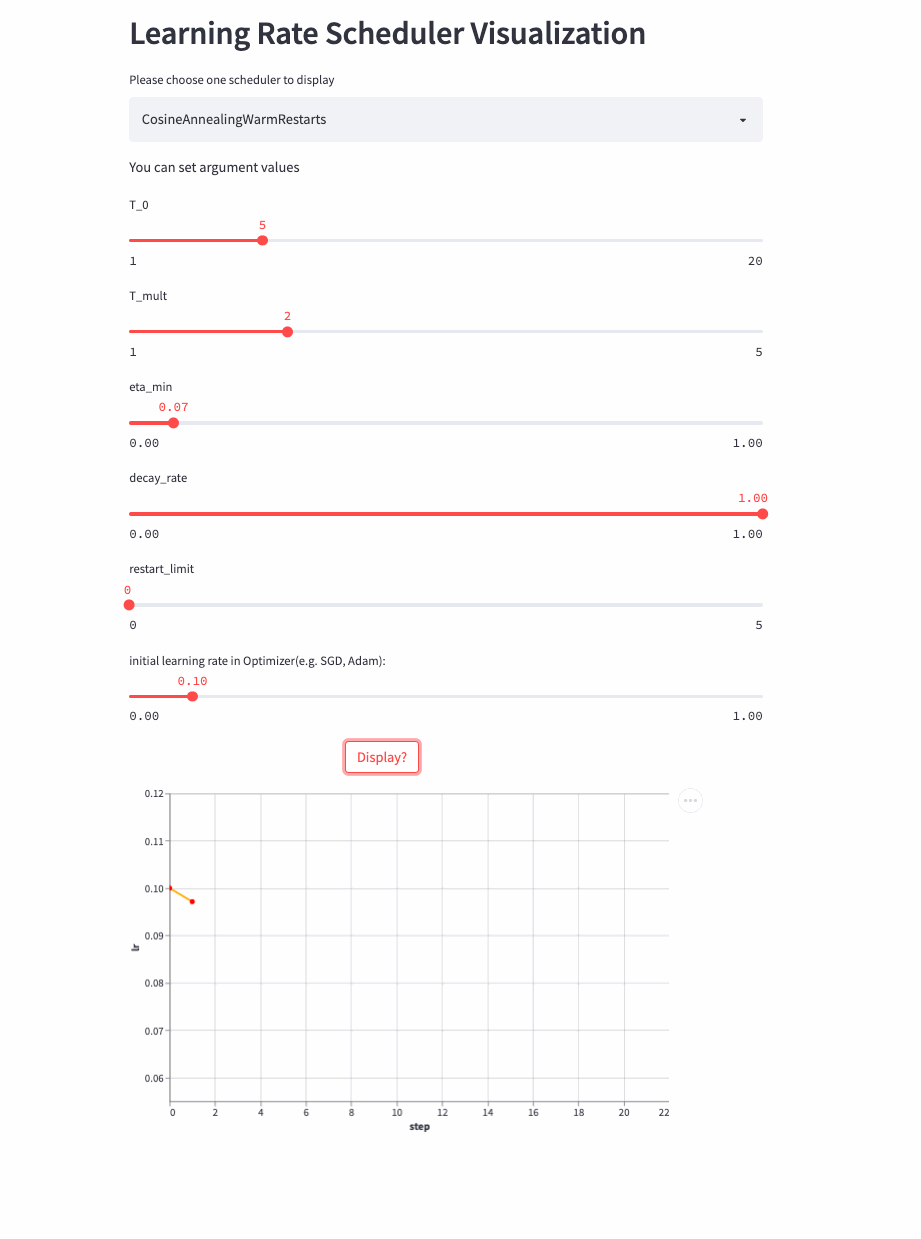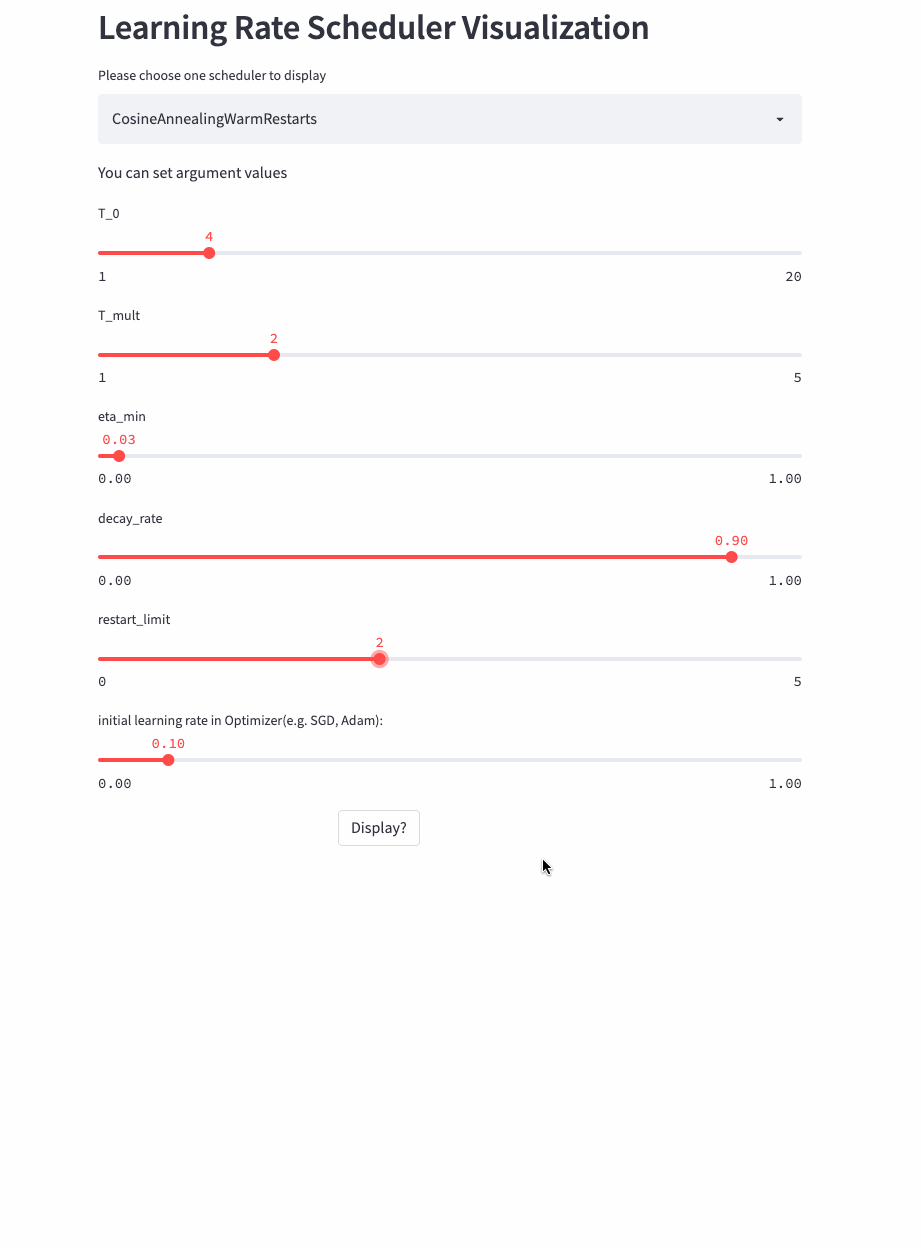### LambdaLR

``oneflow.optim.lr_scheduler.LambdaLR(optimizer, lr_lambda, last_step=-1, verbose=False)``

LambdaLR可以說是最靈活的策略了，因為具體的方法是根據函式lr_lambda來指定的。比如實現Transformer中的Noam Scheduler：

````def rate(step, model_size, factor, warmup):``    """``    we have to default the step to 1 for LambdaLR function``    to avoid zero raising to negative power.``    """``    if step == 0:``        step = 1``    return factor * (``        model_size ** (-0.5) * min(step ** (-0.5), step * warmup ** (-1.5))``    )`

`model = CustomTransformer(...)``optimizer = flow.optim.Adam(``    model.parameters(), lr=1.0, betas=(0.9, 0.98), eps=1e-9``)``lr_scheduler = LambdaLR(``    optimizer=optimizer,``    lr_lambda=lambda step: rate(step, d_model, factor=1, warmup=3000),``)````

### SequentialLR

``oneflow.optim.lr_scheduler.SequentialLR(``    optimizer: Optimizer,``    schedulers: Sequence[LRScheduler],``    milestones: Sequence[int],``    interval_rescaling: Union[Sequence[bool], bool] = False,``    last_step: int = -1,``    verbose: bool = False,``)``

### WarmupLR

``oneflow.optim.lr_scheduler.WarmupLR(``    scheduler_or_optimizer: Union[LRScheduler, Optimizer],``    warmup_factor: float = 1.0 / 3,``    warmup_iters: int = 5,``    warmup_method: str = "linear",``    warmup_prefix: bool = False,``    last_step=-1,``    verbose=False,``)``

WarmupLR是SequentialLR的子類，包含兩個LRScheduler，並且第一個要麼是ConstantLR，要麼是LinearLR。

### ChainedScheduler

``oneflow.optim.lr_scheduler.ChainedScheduler(schedulers)``

``lr ==> LRScheduler_1 ==> LRScheduler_2 ==> ... ==> LRScheduler_N``

### ReduceLROnPlateau

``oneflow.optim.lr_scheduler.ReduceLROnPlateau(``    optimizer,``    mode="min",``    factor=0.1,``    patience=10,``    threshold=1e-4,``    threshold_mode="rel",``    cooldown=0,``    min_lr=0,``    eps=1e-8,``    verbose=False,``)``

``optimizer = flow.optim.SGD(model.parameters(), lr=0.1, momentum=0.9)``scheduler = flow.optim.lr_scheduler.ReduceLROnPlateau(optimizer, 'min')``for epoch in range(10):``    train(...)``    val_loss = validate(...)``    # 注意，該步驟應在validate()之後呼叫。``    scheduler.step(val_loss)``

## 實踐

• https://github.com/basicv8vc/oneflow-cifar100-lr-scheduler

（本文經授權後釋出，原文：

https://zhuanlan.zhihu.com/p/520719314 ）